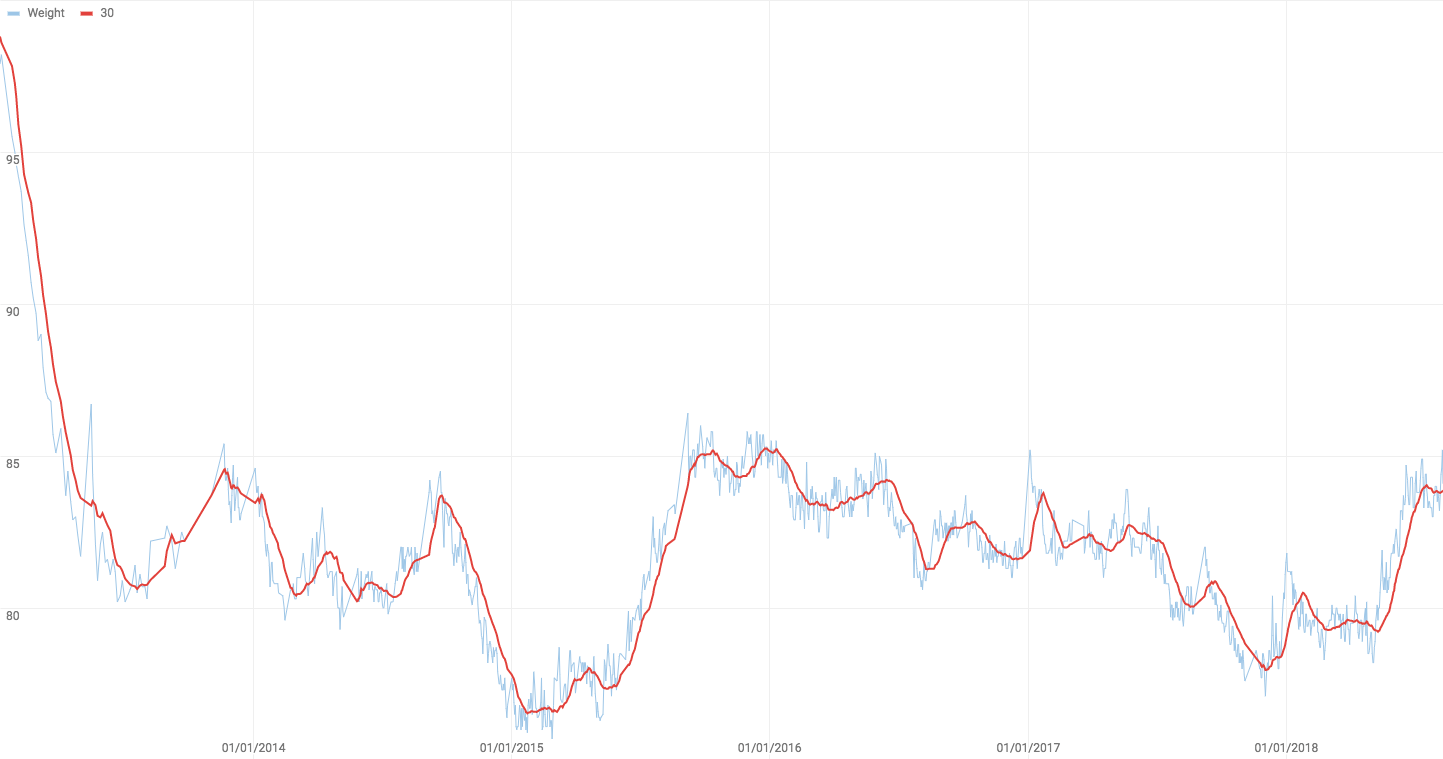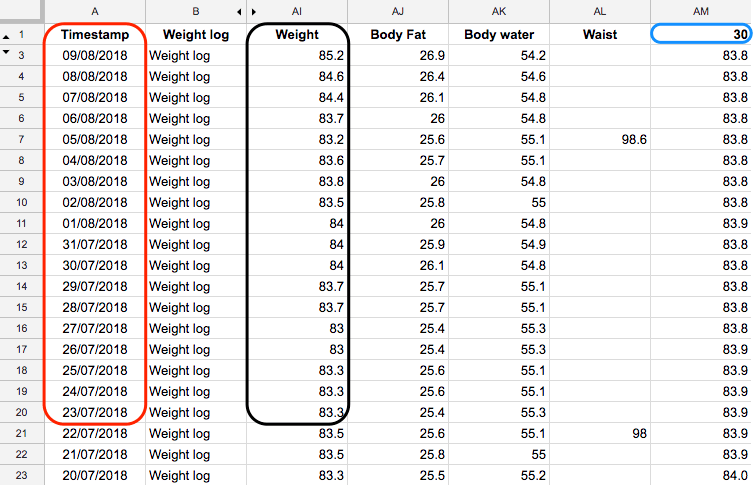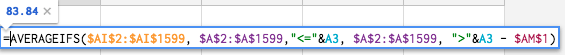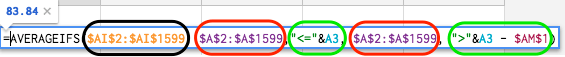# TIL: Moving average with missing periods in Google Sheets

The short answer:

`=AVERAGEIFS(A1:A99, B1:B99,"<="&B2, B1:B99, ">"&B2 - 30)`

Values to be averaged are in A1:A99, corresponding dates are in B1:B99.

What the formula does: average the values in the range – Include a value in calculating average for the current date if:

1. The date for that value is same as or before the date in the current row, and
2. The date for that value is greator than the date X days before the date in the current row (X is 30 in the formula for a 30-day moving average)

The long one:

I have a spreadsheet with my daily weight log. It has occasional missing days – when I didn’t log my weight.

Yesterday, I wanted to chart this data, and wanted to add a moving average to it. Google sheets’ in-built moving average trend line refused to work – either due to the missing data, or due to the number of entries. So I added a column to the sheet with the calculated (trailing) moving average weight.My weight and the 30-day moving average

I’ve never before had to calculate moving average over a non-consecutive data set. So, in case I forget, I’m noting it down here for later…

The table was setup as below (please don’t judge me on that weight – I’m struggling!)The sheet with data entries

The formula used was:The moving average formula

Broken down into bits, the formula is:The moving average formula – parts

`averageifs`’ provides the average of a cells in range where all corresponding conditions are met. The inputs to the formula are:

1. The range with values to average. In my case it was the column with weight values – circled in black
2. The range over which to test the first condition. In this case it was the column with dates – first red circle
3. The condition that the first range has to meet – first green circle.
• – I average the rows where date is same as, or before the date of this row. i.e. ‘`<= row_date_cell`
4. The range over which to test the second condition. It was again the column with dates – second red circle
5. The condition that the second range has to meet – second green circle.
• – I average the rows where date is greator than the date that is `X` days before the date in this row, i.e. ‘`> row_date_cell - X`
• `X` defines how many days of data to average. In the graph above, I use a 30-day moving average. By changing the value in cell `AM1`, I could easily view the same graph with other values – a 7 day moving average, for instance.

Update: Spreadsheet with working sample.

Previously: Conditional sum product in Google Sheets

## 3 thoughts on “TIL: Moving average with missing periods in Google Sheets”

1.Sebbe says:

is it possible to get a link to the spreadsheet?

1.Aditya says:

Hey, thanks for your comment. I’ve added a sample spreadsheet link at bottom of the post.

2.Nicholas Barry says:

This is really helpful! Thanks for sharing this technique. One way you can simplify the formula a little bit, and use AVERAGEIF instead of AVERAGEIFS is to use an anchored data range (I don’t know if that’s the official term) to avoid picking up data in the future. This only works if your logs are in date order already, but they probably are. So you could write:

=AVERAGEIF(A\$2:A2, “>”&A2 – 30, B\$2:B2)

I’m assuming that row 1 is a header row, so I’m starting with data in row 2. As you copy that cell down, the A2 (and B2) parts will update, while the anchored A\$2 and B\$2 won’t update. So the average cell will never even look at rows in the future (with higher row numbers).

This site uses Akismet to reduce spam. Learn how your comment data is processed.